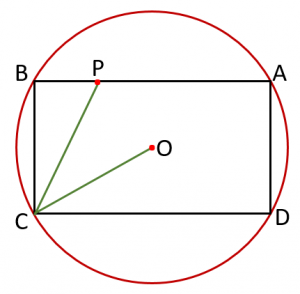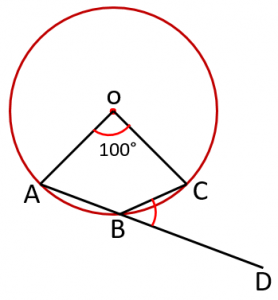Monday, September 27, 2021
Home > geometry > SSC CGL Geometry Circle Set 3

# SSC CGL Geometry Circle Set 3

SSC CGL CHSL Geometry
Tangent to Circle Questions Sets
Set 1Set 2Set 3Set 4

SSC CGL Geometry Circle Set 3 : Ques No 1

ABCD is a cyclic trapezium with AB || DC and AB as the diameter. If ∠CAB = 30 degrees, then ∠ADC is

Options:

A. 120 degrees

B. 30 degrees

C. 150 degrees

D. 60 degrees

Solution:

SSC CGL Geometry Circle Set 3 : Ques No 2

Length of a chord PQ in a circle is 18 cm. AB is the perpendicular bisector of PQ at M and intersects the circle at A and B. If MB = 3 cm, then the length of AB is

Options:

A. 25 cm

B. 27 cm

C. 28 cm

D. 30 cm

Solution:

SSC CGL Geometry Circle Set 3 : Ques No 3

Two parallel chords are drawn in a circle of diameter 30 cm. The length of one chord is 24 cm and the distance between the chords is 21 cm. The length of the other chord is then

Options:

A. 10 cm

B. 12 cm

C. 18 cm

D. 15 cm

Solution:

SSC CGL Geometry Circle Set 3 : Ques No 4

If three non-collinear points A, B and C lie on the periphery of a circle so that AB = AC = BC = 3 cm, then the radius of the circle (in cm) is

Options:

A. √3

B. 1/√3

C. √3/2

D. 2/√3

Solution:

SSC CGL Geometry Circle Set 3 : Ques No 5

In the figure below, ABCD is a rectangle inscribed inside the circle with centre at O so that the ratio of areas of the rectangle to the circle is √3 : π. Line segment CP intersects AB at P. If ∠OCD = ∠BCP, the ratio of BP : BC equalsOptions:

A. 1 : √2

B. 1 : √3

C. 1 : 2

D. 1 : 2√3

Solution:

SSC CGL Geometry Circle Set 3 : Ques No 6

In the figure below, O is the centre of the circle and A, B, C and D are the four points on the circumference of the circle.  Line segments AD and BC intersect at Q so that ∠AQB = 100 degrees, while segments CA and DB extended meet at a point P outside the circle and ∠CPD = 60 degrees. Find the value of ∠AOBOptions:

A. 60 degrees

B. 40 degrees

C. 50 degrees

D. 55 degrees

Solution:SSC CGL Geometry Circle Set 3 : Ques No 7

If two equal circles are such that the centre of one lies on the periphery of the other, then the ratio of the common chord to the radius of any of the circle is

Options:

A. √3 : 2

B. √3 : 1

C. √5 : 1

D. 1 : √3

Solution:

SSC CGL Geometry Circle Set 3 : Ques No 8

In the figure below, an arc ABC of a circle subtends an angle of 100 degrees at the center O. If AB is extended to a point D outside the circle, the value of ∠CBD isOptions:

A. 40 degrees

B. 140 degrees

C. 50 degrees

D. 130 degrees

Solution:

SSC CGL Geometry Circle Set 3 : Ques No 9

In the figure below, ∠AEB = 35 degrees and ∠ADF = 75 degrees. The value of ∠AFD isOptions:

A. 65 degrees

B. 45 degrees

C. 35 degrees

D. 55 degrees

Solution:

SSC CGL Geometry Circle Set 3 : Ques No 10

In the figure below, O is the center of the circle with ∠COD = 106 degrees and AC as the diameter. Then the value of ∠ABD isOptions:

A. 55 degrees

B. 40 degrees

C. 37 degrees

D. 43 degrees